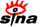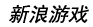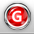下载新浪UT Game　客户端高速下载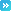推荐：全球新网游 | 网页游戏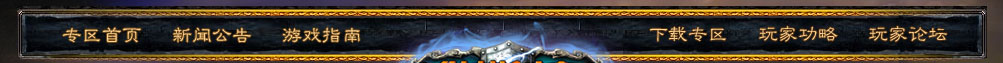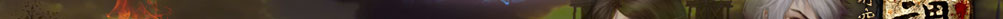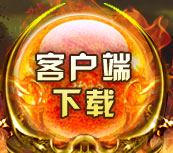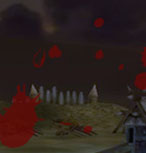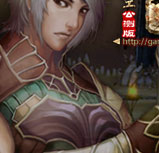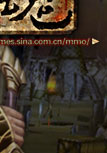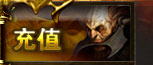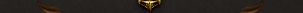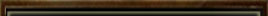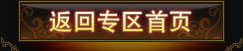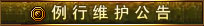为了保证游戏运行的稳定性及提升整体服务质量，《纵横时空》游戏全部服务器将每周二凌晨4：00-12：00进行例行停机维护， 停机期间给您带来的不便，敬请谅解。祝大家游戏愉快！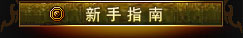登录游戏 创建角色 界面说明 基本操作 快 捷 键 角色属性 系统设置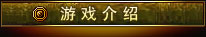背景故事 世界介绍 两大阵营 五大宗派 配置说明 下载安装 收费充值 Vista系统启动指南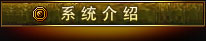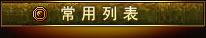游戏问题联系电话：            010-84799696 充值问题联系电话：客服电话：010-52926702/03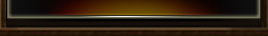隐匿之林

 任务名称 接受等级 给予任务NPC 阵营需求 任务类型 清剿树魁 >=20 古战阳 新洛 主线任务 图腾木柱 >=19 旗飘展 新洛 主线任务 探访 >=20 旗飘展 新洛 主线任务 强化结晶 >=20 旗飘展 新洛 主线任务 死尸之村 >=20 军刀 新洛 主线任务 夺回血样 >=20 灰惊剑 新洛 主线任务 幽魂的惨叫 >=21 灰惊剑 新洛 主线任务 紧急求援I >=21 灰惊剑 新洛 主线任务 紧急求援II >=21 军刀 新洛 主线任务 告戒I >=20 旗飘展 新洛 主线任务 灰惊剑的建议 >=22 灰惊剑 新洛 主线任务 旗飘展的建议 >=23 旗飘展 新洛 主线任务 告戒II >=24 旗飘展 新洛 主线任务 采集黑油草 >=24 板平燕 新洛 主线任务 迈向天涯海角 >=25 板平燕 新洛 主线任务 前往月沐林 >=18 洛青剑 正仪 主线任务 木妖危机I >=19 卡坡 正仪 主线任务 击杀木妖 >=19 卡坡 正仪 主线任务 木妖危机II >=19 卡坡 正仪 主线任务 突击围剿I >=19 卡坡 正仪 主线任务 遁影宅迷乱I >=20 卡坡 正仪 主线任务 遁影宅迷乱II >=20 高塔柱 正仪 主线任务 遁影宅迷乱III >=21 高塔柱 正仪 主线任务 遁影宅迷乱VI >=21 卡坡 正仪 主线任务 全面进攻 >=22 高塔柱 正仪 主线任务 高塔柱的建议 >=22 高塔柱 正仪 主线任务 庄中阳的建议 >=22 庄中阳 正仪 主线任务 抢回补给 >=23 于音 正仪 主线任务 消灭兽人I >=23 于音 正仪 主线任务 神奇的草 >=24 于音 正仪 主线任务 于音的嘱托 >=24 于音 正仪 主线任务 走向天涯海角 >=25 于音 正仪 主线任务 互相认识 >=20 庄中阳 正仪 主线任务 神奇传送 >=20 孔数银 正仪 主线任务 回去领命 >=20 月沐林传送员 正仪 主线任务 前去报道 >=20 庄中阳 正仪 主线任务 历练 >=20 卡坡 正仪 主线任务 遁影宅迷乱IV >=23 卡坡 正仪 主线任务 遁影宅迷乱V >=23 庄中阳 正仪 主线任务 做准备 >=20 庄中阳 正仪 主线任务 反击 >=19 旗飘展 新洛 支线任务 驻守边疆 >=19 旗飘展 新洛 支线任务 危机四伏 >=19 张恒 新洛 支线任务 鬼魂传说 >=22 张恒 新洛 支线任务 巡逻兵李坚 >=23 旗飘展 新洛 支线任务 病痛 >=21 李坚 新洛 支线任务 寻找药材 >=21 尚离 新洛 支线任务 送药 >=21 尚离 新洛 支线任务 树魁之王(jy) >=21 军刀 新洛 支线任务 旧宅鬼影 >=23 老者 新洛 支线任务 招魂使者 >=24 老者 新洛 支线任务 超渡亡灵(jy) >=26 老者 新洛 支线任务 缉拿真凶(jy) >=24 老者 新洛 支线任务 密信事件I >=20 支线任务 密信事件II >=20 尚离 支线任务 密信事件III(精英) >=20 尚离 支线任务 交锋(jy) >=23 灰惊剑 新洛 支线任务 夺回补给 >=24 板平燕 新洛 支线任务 圣火邪教(jy) >=26 板平燕 新洛 支线任务 老者的嘱托 >=27 老者 新洛 支线任务 魂引符石 >=25 尚离 新洛 支线任务 给妖狐一个教训 >=20 井云 正仪 支线任务 迎接冬季 >=20 井云 正仪 支线任务 漂亮的羽毛 >=20 孔数银 正仪 支线任务 小虎的玩具I >=20 小虎 正仪 支线任务 小虎的玩具II >=21 小虎 正仪 支线任务 小虎的玩具III >=21 孔数银 正仪 支线任务 枯木成精(jy) >=19 庄中阳 正仪 支线任务 村民的委托 >=19 孔数银 正仪 支线任务 慰劳士兵 >=21 高塔柱 正仪 支线任务 消灭木妖王(jy) >=21 卡坡 正仪 支线任务 将军的关爱 >=20 庄中阳 正仪 支线任务 盔甲 >=24 孔数银 正仪 支线任务 被抢走的包裹 >=22 井云 正仪 支线任务 神秘的玄武典籍(jy) >=23 高塔柱 正仪 支线任务 清剿行动 >=23 高塔柱 正仪 支线任务 狼子野心 >=22 庄中阳 正仪 支线任务 消灭盘蛇魔王(jy) >=24 高塔柱 正仪 支线任务 保护草场 >=19 老酒鬼 正仪 支线任务 牧民的委托(jy) >=26 牧民 正仪 支线任务 嗜血飞蝠 >=23 老酒鬼 正仪 支线任务 神奇的神木草 >=23 井云 正仪 支线任务 士兵的修炼I >=19 庄中阳 正仪 支线任务 士兵的修炼II >=19 庄中阳 正仪 支线任务 士兵的修炼III >=19 庄中阳 正仪 支线任务 领地 >=20 古战阳 新洛 阵营任务 报到 >=20 古战阳 新洛 阵营任务 探查小径 >=20 旗飘展 新洛 阵营任务 采药人 >=20 板平燕 新洛 阵营任务 清除兽人 >=20 朱老伯 新洛 阵营任务 采集兰尾草 >=20 朱老伯 新洛 阵营任务 兽人的兵器 >=20 朱老伯 新洛 阵营任务 汇报军情 >=20 朱老伯 新洛 阵营任务 递送请战书 >=20 板平燕 新洛 阵营任务 领地之争 >=20 洛青剑 正仪 阵营任务 将军的命令 >=20 洛青剑 正仪 阵营任务 探路 >=20 庄中阳 正仪 阵营任务 联络线人 >=20 于音 正仪 阵营任务 清除隐患 >=20 李航 正仪 阵营任务 李航的委托 >=20 李航 正仪 阵营任务 神奇药丸 >=20 李航 正仪 阵营任务 汇报战情 >=20 李航 正仪 阵营任务 申请战斗 >=20 于音 正仪 阵营任务 夺回圣物 >=10 旗飘展 新洛 活动任务 夺回圣物 >=10 庄中阳 正仪 活动任务 寻找斥候 >=19 高塔柱 正仪 支线任务 找回情报 >=19 斥候 正仪 支线任务 暗魂的走狗 >=19 斥候 正仪 支线任务 带回情报 >=19 斥候 正仪 支线任务 怪异事件 >=19 高塔柱 正仪 支线任务 恩怨 >=19 于音 正仪 支线任务 计划实施 >=19 于音 正仪 支线任务 完美计划 >=19 于音 正仪 支线任务 突击围剿II >=19 卡坡 正仪 支线任务 突击围剿III >=19 卡坡 正仪 支线任务 消灭兽人II >=19 于音 正仪 支线任务 净化水源I >=19 老者 新洛 支线任务 净化水源II >=19 宁渊 新洛 支线任务 净化水源III >=19 宁渊 新洛 支线任务 净化水源IV >=19 尚离 新洛 支线任务 冒险家李航I >=19 尚离 新洛 支线任务 冒险家李航II >=19 李航 新洛 支线任务 冒险家李航III >=19 李航 新洛 支线任务 全面攻击I >=19 旗飘展 新洛 支线任务 【返回首页】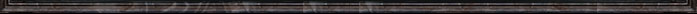留言 更多>>
 本新闻留言共条 显示 条
 登录名： 　密码： 　 匿名发表